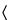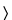Potassium Acetate
(poe tas' ee um as' e tate).

C2H3KO2 98.14
Acetic acid, potassium salt;
Potassium acetate[127-08-2].
DEFINITION
Potassium Acetate contains NLT 99.0% and NMT 100.5% of C2H3KO2, calculated on the dried basis.
IDENTIFICATION
Sample solution:  100 mg/mL
Acceptance criteria:  Meets the requirements
Sample solution:  100 mg/mL
Acceptance criteria:  Meets the requirements
ASSAY
•  Procedure
Sample:  200 mg of Potassium Acetate, previously dried
Analysis:  Dissolve the Sample in 25 mL of glacial acetic acid, add 2 drops of crystal violet TS, and titrate with 0.1 N perchloric acid VS to a green endpoint. Perform a blank determination, and make any necessary correction (see Titrimetry541). Each mL of 0.1 N perchloric acid is equivalent to 9.814 mg of potassium acetate (C2H3KO2).
Acceptance criteria:  99.0%–100.5% on the dried basis
IMPURITIES
Test preparation:  Dissolve 1 g of Potassium Acetate in 10 mL of water, add 3.0 mL of glacial acetic acid, and dilute with water to 25 mL. Adjust with glacial acetic acid to a pH of 3.8–4.0, measured with a pH meter.
Monitor preparation:  Prepare as directed for the Test preparation, 2.0 mL of Standard Lead Solution being added.
Acceptance criteria:  NMT 20 ppm
•  Limit of Sodium
Solution A:  100 mg/mL of potassium chloride in water
Standard stock solution:  Transfer 127.1 mg of sodium chloride, previously dried at 105for 2 h into a 500-mL volumetric flask, add water to volume, and mix. Transfer 10.0 mL of this solution to a 100-mL volumetric flask, dilute with water to volume, and mix.
Standard solutions:  Transfer 2.0, 5.0, and 10.0 mL of the Standard stock solution into separate 100-mL volumetric flasks, add 10.0 mL of Solution A to each flask, and dilute with water to volume. These Standard solutions contain 0.2, 0.5, and 1.0 µg/mL of sodium, respectively. [Note—Concentrations of sodium in the Standard solutions may be modified to fit the linear or working range of the atomic absorption spectrophotometer. ]
Sample solution:  Transfer about 0.2 g of Potassium Acetate into a 100-mL volumetric flask containing about 50 mL of water, and swirl to dissolve. Add 10.0 mL of Solution A, dilute with water to volume, and mix. [Note—The concentration of Potassium Acetate in the Sample solution may be modified by using a different quantity or by further dilution to bring the absorption response within the range of responses from the Standard solutions. ]
Instrumental conditions
Mode:  Atomic absorption spectrophotometry
Analytical wavelength:  Sodium emission line at 589 nm
Lamp:  Sodium hollow-cathode
Flame:  Oxidizing air–acetylene
Blank solution:  Transfer 10.0 mL of Solution A into a 100-mL volumetric flask, dilute with water to volume, and mix.
Analysis
Samples:  Standard solutions, Sample solution, and Blank solution
Determine the absorbances of the Standard solutions and the Sample solution, using the Blank solution to zero the instrument. Plot the absorbances of the Standard solutions versus the concentration, in µg/mL, of sodium, and draw the straight line best fitting the plotted points. From the graph so obtained, determine the concentration, C, in µg/mL, of sodium in the Sample solution.
Calculate the percentage of sodium in the portion of Potassium Acetate taken:
Result = (C/W) × (V/F) × 100
 C = = concentration of the Sample solution, determined from the graph (µg/mL) W = = weight of Potassium Acetate taken to prepare the Sample solution (g) V = = final volume of the Sample solution, taking into account any dilution necessary (mL) F = = conversion factor (1,000,000 µg/g)
Acceptance criteria:  NMT 0.03%
SPECIFIC TESTS
Sample solution:  50 mg/mL
Acceptance criteria:  7.5–8.5
Dry a sample at 150for 2 h: it loses NMT 1.0% of its weight.